The Bead Unbaffled - An Abacus Manual

###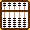Long Division Techniques - Chinese Suan Pan

This powerful technique was first shown to me by Torsten Reincke. It's the second chapter in how to solve problems of division using traditional Chinese techniques. Before continuing it's suggested that one should have an understanding of the techniques and rules that govern Chinese short division .

This method of long division uses a traditional 2:5 bead suan pan. Over the years many have speculated on why these Chinese instruments carry extra beads; these techniques may shed some light on the question.

1. The Extra Bead Technique which uses the top most heaven bead. It has a value of 5. When this technique is used the two top most beads have a value of 5 + 5 = 10 (Fig.2 in the second example - the extra bead is placed on rod F.)

2. The Suspended Bead Technique which also uses the top most heaven bead but in this case it is set  ½ way down the rod so that the bead neither touches the frame above nor the bead below. It has a value of 10. When this technique is used the two top most beads have a value of 10 + 5 = 15 (Fig.5 in the third example - the suspended bead is placed on rod H.)

* It should be noted that as with any method for solving problems of division on an abacus, there will be instances where the abacus operator will have to revise a quotient answer either up or down in order to come to the correct result. Although remarkably good, this technique is no exception.

##### Division And Shifting the Unit Rod If the Divisor is.... 1.003..... one whole number - unit rod shifts 1 rod to the left. 45.03..... two whole numbers - unit rod shifts 2 rods to the left. 0.75....... no whole numbers, no trailing zeros, unit rod does *not* shift. 0.0125... one trailing zero - unit rod shifts 1 rod to the right. 0.0036... two trailing zeros - unit rod shifts 2 rods to the right...etc.
 Suan Pan and the Decimal - Division: Shifting

Below are three examples for this technique. Even though the first two examples have decimal answers each problem is quite straight forward and demonstrates the method very well. In fact shifting the unit rod in order to find the correct decimal answer is very easy when using this suan pan technique. One only has to take into account either the whole numbers or the trailing zeros in the divisor in order to find the correct decimal answer.

For our purposes it will be enough to solve each of the first two problems to four decimal places with a remainder at the end.

Example 1: 290 ÷ 47 = 6.1702  ==> with remainder 0.0006 (see Fig. 8)

This example uses the rules;

a) rule 2/4 = 5
b) rule 4/4 = forward 1
c) rule 3/4 = 7 plus 2
d) rule 1/4 = 2 plus 2

Step 1: Choose rod G as the unit rod. Set the dividend 290 on rods EFG and divisor 47 on AB. The divisor has two whole numbers therefore the unit rod shifts two rods to the left brining us to rod E.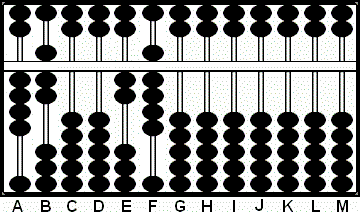Fig. 1 `Step 1` ```A B C D E F G H I J K L M       4 7 0 0 2 9 0 0 0 0 0 0 0 Step 1```

Step 2:
Compare 4 on A and 2 on E.  Follow ==> rule: 2/4 = 5 <== The first number in the quotient answer will be 5. Place 5 on rod E. Multiply 5 on E by 7 on B and subtract the product 35 from rods FG. With 55 remaining on FG it's evident that we can subtract another 47 from rods FG. (Fig.2)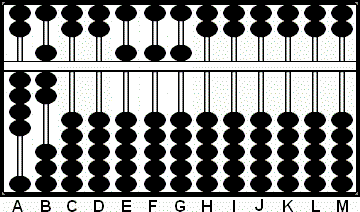Fig. 2 `Step 2` ```A B C D E F G H I J K L M       4 7 0 0 2 9 0 0 0 0 0 0 0 (5) - 3 5 Step 2 4 7 0 0 5 5 5 0 0 0 0 0 0```

Step 3:
Revise the answer: add 1 to the quotient on E and subtract a further 47 from rods FG. This leaves quotient 6 on E and remainder 8 on G. (Fig. 3)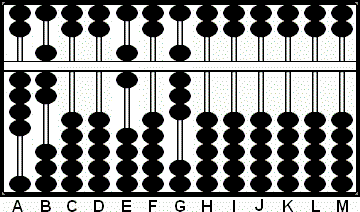Fig. 3 `Step 3` ```A B C D E F G H I J K L M       4 7 0 0 5 5 5 0 0 0 0 0 0 + 1 - 4 7 Step 3 4 7 0 0 6 0 8 0 0 0 0 0 0```

Step 4:
Compare 4 on A with 8 on G. If we forward 2 there won't be anything left to continue therefore follow rule ==> 4/4 = forward 1 <=== The next number in the quotient answer will be 1. Forward 1 to F and subtract 47 from rods GH. This leaves interim quotient 61 on EF and remainder 33 on rods GH. (Fig.4)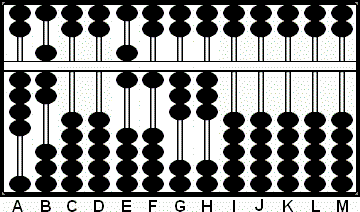Fig. 4 `Step 4` ```A B C D E F G H I J K L M       4 7 0 0 6 0 8 0 0 0 0 0 0 + 1 - 4 7 Step 4 4 7 0 0 6 1 3 3 0 0 0 0 0```

Step 5:
Compare 4 on A and 3 on G.  Follow rule ==> 3/4 = 7 plus 2 <== The next number in our quotient will be 7. Place 7 on rod G and add 2 to rod H. (Fig.5)Fig. 5 `Step 5` ```A B C D E F G H I J K L M       4 7 0 0 6 1 3 3 0 0 0 0 0 (7) + 2 Step 5 4 7 0 0 6 1 7 5 0 0 0 0 0```

Step 6:
Multiply 7 on G by 7 on rod B and subtract the product 49 from rods HI. This leaves interim quotient 617 on EFG and remainder 1 on rod I. (Fig. 6)Fig. 6 `Step 6` ```A B C D E F G H I J K L M       4 7 0 0 6 1 7 5 0 0 0 0 0 - 4 9 Step 6 4 7 0 0 6 1 7 0 1 0 0 0 0```

Step 7:
Next compare 4 on A and 1 on I.  Follow rule ==> 1/4 = 2 plus 2 <== The next number in the quotient will be 2. Place 2 on rod I and add the 2 on J. (Fig. 7)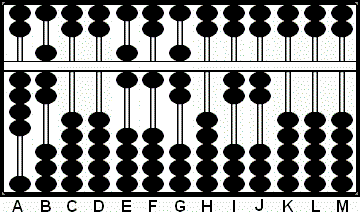Fig. 7 `Step 7` ```A B C D E F G H I J K L M       4 7 0 0 6 1 7 0 1 0 0 0 0 (2) + 2 Step 7 4 7 0 0 6 1 7 0 2 2 0 0 0```

Step 8:
Multiply 2 on I by 7 on B and subtract the product 14 from rods JK. This is as far as we'll go in this problem. Because we shifted the unit rod to E in the first step the answer so far is 6.1702 on rods E - I and the remainder 6 on rod K. (Fig. 8)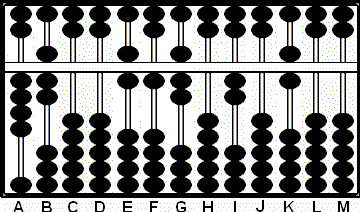Fig. 8 `Step 8` ```A B C D E F G H I J K L M       4 7 0 0 6 1 7 0 2 2 0 0 0 - 1 4 Step 8 4 7 0 0 6 1 7 0 2 0 6 0 0```

Example 2:  28 ÷ 73 = 0.3835

==> with remainder 0.0045 (see Fig. 11)

This example uses the rules;

a) rule 2/7 = 2 plus 6
b) rule 6/7 = 8 plus 4
c) rule 2/7 = 2 plus 6
d) rule 4/7 = 5 plus 5

Step 1: Choose rod F as the unit rod. Set the dividend 28 on rods EF and divisor 73 on AB. The divisor has two whole numbers therefore the unit rod shifts two to the left brining us to rod D.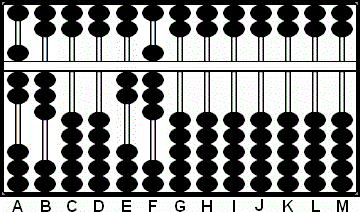Fig. 1 `Step 1` ```A B C D E F G H I J K L M       7 3 0 0 2 8 0 0 0 0 0 0 0 Step 1 ```

Step 2:
Compare 7 on A and 2 on E.  Follow rule  ==> 2/7 = 2 plus 6 <== The first number in our quotient answer will be 2. Place 2 on rod E and add 6 to rod F. With 14 on rod F we need to revise the answer. (Fig.2)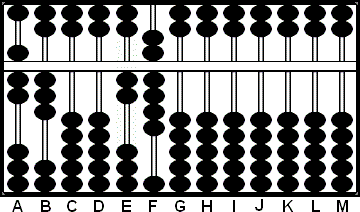Fig. 2 `Step 2` ```A B C D E F G H I J K L M       7 3 0 0 2 8 0 0 0 0 0 0 0 (2) + 6 Step 2 7 3 0 0 2'14'0 0 0 0 0 0 0 ```

Step 3:
Revise the answer: add 1 to the quotient on rod E and subtract a further 7 from F leaving 37. (Fig. 3)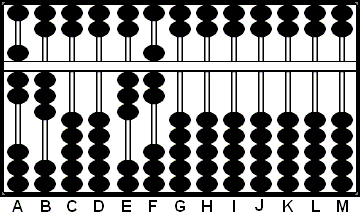Fig. 3 `Step 3` ```A B C D E F G H I J K L M       7 3 0 0 2'14'0 0 0 0 0 0 0 + 1 - 7 Step 3 7 3 0 0 3 7 0 0 0 0 0 0 0 ```

Step 4: Now multiply 3 on rod E by 3 on B and subtract the product 09 from rods FG. This leaves the first number in our quotient 3 on rod E and the remainder 61 on FG. (Fig. 4)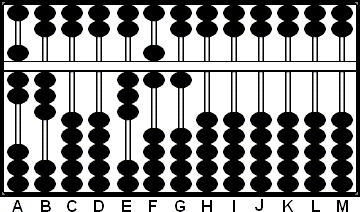Fig. 4 `Step 4` ```A B C D E F G H I J K L M       7 3 0 0 3 7 0 0 0 0 0 0 0 - 0 9 Step 4 7 3 0 0 3 6 1 0 0 0 0 0 0 ```

Step 5: Now compare 7 on A with 6 on F. Follow rule ==> 6/7 = 8 plus 4 <== The next number in our quotient will be 8. Place 8 on rod F and add 4 to G.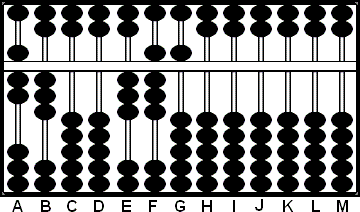Fig. 5 `Step 5` ```A B C D E F G H I J K L M       7 3 0 0 3 6 1 0 0 0 0 0 0 (8) + 4 Step 5 7 3 0 0 3 8 5 0 0 0 0 0 0 ```

Step 6: Now multiply 8 on F by 3 on B and subtract the product 24 from rods GH. This leaves the interim quotient 38 on EF and the remainder 26 on GH. (Fig. 6)Fig. 6 `Step 6` ```A B C D E F G H I J K L M       7 3 0 0 3 8 5 0 0 0 0 0 0 - 2 4 Step 6 7 3 0 0 3 8 2 6 0 0 0 0 0 ```

Step 7: Compare 7 on A with 2 on G. Follow the rule ==> 2/7 = 2 plus 6 <== The next number in our quotient will be 2. Place 2 on G and add 6 to H. With 12 on rod H we need to revise. (Fig. 7)Fig. 7 `Step 7` ```A B C D E F G H I J K L M       7 3 0 0 3 8 2 6 0 0 0 0 0 (2) + 6 Step 7 7 3 0 0 3 8 2'12'0 0 0 0 0 ```

Step 8: Revise the answer: add 1 to the quotient on G and subtract a further 7 from H. (Fig. 8)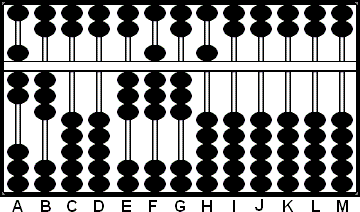Fig. 8 `Step 8` ```A B C D E F G H I J K L M       7 3 0 0 3 8 2'12'0 0 0 0 0 + 1 - 7 Step 8 7 3 0 0 3 8 3 5 0 0 0 0 0 ```

Step 9: Now multiply 3 on G with 3 on B and subtract 09 from rods HI. This leaves the interim quotient 383 on EFG and the remainder 41 on rods HI. (Fig. 9)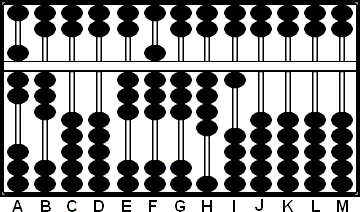Fig. 9 `Step 9` ```A B C D E F G H I J K L M       7 3 0 0 3 8 3 5 0 0 0 0 0 - 0 9 Step 9 7 3 0 0 3 8 3 4 1 0 0 0 0 ```

Step 10: Compare 7 on A with 4 on H. Follow the rule ==> 4/7 = 5 plus 5 <== The next number in our quotient will be 5. Place 5 on H and add 5 to rod I. (Fig. 10)Fig. 10 `Step 9` ```A B C D E F G H I J K L M       7 3 0 0 3 8 3 4 1 0 0 0 0 (5) + 5 Step 10 7 3 0 0 3 8 3 5 6 0 0 0 0 ```

Step 11: Now multiply 5 on H with 3 on B and subtract 15 from rods IJ. This is as far as we'll go in this problem. Because we shifted the unit rod to rod D in the first step the answer so far is 0.3835 on rods D - H and the remainder 45 on rods IJ. (Fig. 11)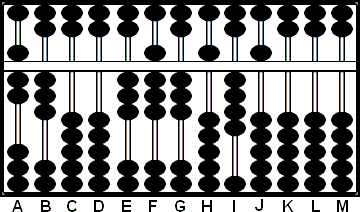Fig. 11 `Step 9` ```A B C D E F G H I J K L M       7 3 0 0 3 8 3 5 6 0 0 0 0 - 1 5 Step 11 7 3 0 0 3 8 3 5 4 5 0 0 0 ```

Example 3:  58174 ÷ 986 = 59

This example uses the rules;

a) rule 5 /9= 5 plus5
b) rule 8 /9= 8 plus 8
c) rule 9 /9= forward 1

Step 1:  Choose rod J as the unit rod. Set the dividend 58174 on rods FGHIJ and the divisor 986 on ABC.  The divisor has three whole numbers therefore the unit rod shifts three rods to the left brining us to rod G. (Fig.1)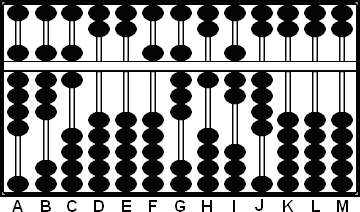Fig. 1 `Step 1` ```A B C D E F G H I J K L M       9 8 6 0 0 5 8 1 7 4 0 0 0 ```

Step 2:
Follow rule ==> 5 /9= 5 plus 5 <== place the quotient 5 on rod F and add 5 to G, leaving 5 "13" 1 7 4 on FGHIJ. (Note: the Extra Bead Technique allows for 13 to be placed on rod G.) (Fig.2)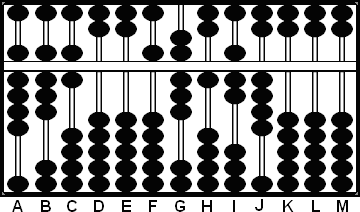Fig. 2 `Step 2` ```A B C D E F G H I J K L M       9 8 6 0 0 5 8 1 7 4 0 0 0 plus 5 Step 2 9 8 6 0 0 5'13'1 7 4 0 0 0 ```

2a:
Multiply 5 on F by 8 on B. Subtract the product 40 from rods GH, leaving, 59174 on rods F - J. (Fig.3)Fig. 3 `Step 2a` ```A B C D E F G H I J K L M       9 8 6 0 0 5'13'1 7 4 0 0 0 - 4 0 Step 2a 9 8 6 0 0 5 9 1 7 4 0 0 0 ```

2b:
Multiply 5 on F by 6 on C. Subtract the product 30 from rods HI, leaving the interim quotient 5 on F and the remainder 8874 on rods G - J. (Fig.4)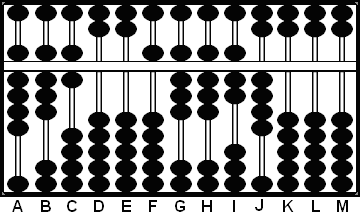Fig. 4 `Step 2b` ```A B C D E F G H I J K L M       9 8 6 0 0 5 9 1 7 4 0 0 0 - 3 0 Step 2b 9 8 6 0 0 5 8 8 7 4 0 0 0 ```

Step 3:
Follow rule ==> 8 /9= 8 plus 8 <== place the quotient 8 on G and add the remainder 8 on H.  (Note: Since rod H already carries a large number we don't have 8 available. This is where we'll use  *The Suspended Bead Technique*  which counts for 10.)  Slide the top most bead ½ way down on rod H for a value of 10 then subtract 2 giving us the required 8. This  leaves 5 8 "16" 7 4 on rods FGHIJ. (Fig.5)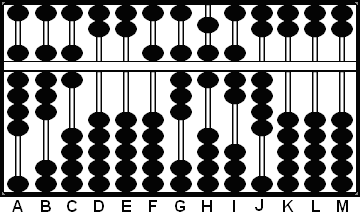Fig. 5 `Step 3` ```A B C D E F G H I J K L M       9 8 6 0 0 5 8 8 7 4 0 0 0 suspended '10' - 2 Step 3 9 8 6 0 0 5 8'16'7 4 0 0 0 ```

3a:
Multiply the 8 on G by the 8 on B and subtract the product 64 from rods HI, leaving 5 8 "10" 3 4 on FGHIJ. (Fig.6)  ***see a variation below***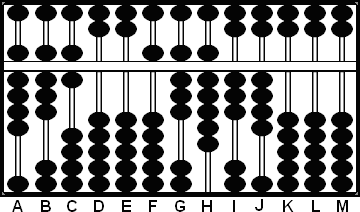Fig. 6 `Step 3a` ```A B C D E F G H I J K L M       9 8 6 0 0 5 8'16'7 4 0 0 0 - 6 4 Step 3a 9 8 6 0 0 5 8'10'3 4 0 0 0 ```

3b:
Multiply the 8 on G by the 6 on C and subtract the product 48 from rods IJ. With 986 remaining on rods HIJ we have to revise the answer. (Fig.7)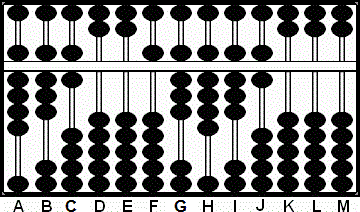Fig. 7 `Step 3b` ```A B C D E F G H I J K L M       9 8 6 0 0 5 8'10'3 4 0 0 0 - 4 8 Step 3b 9 8 6 0 0 5 8 9 8 6 0 0 0 ```

Revise the answer: Add 1 to 8 on G and subtract a further 986 from rods HIJ. This leaves 59 on rods FG which is the answer. (Fig.8)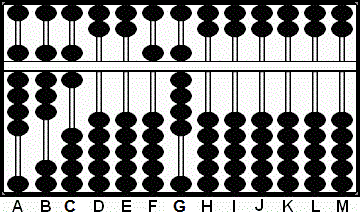Fig. 8 `Step 3c` ```A B C D E F G H I J K L M       9 8 6 0 0 5 8 9 8 6 0 0 0 revise + 1 - 9 8 6 Step 3c 9 8 6 0 0 5 9 0 0 0 0 0 0 ```

***
In the above step 3a one could have exercised the option to do a revision using  rule  ==> 9 /9= forward 1 <==  thereby changing the 8 on rod G to a 9. Multiplying the revised 9 on G by the 8 on B followed by the 6 on C and subtracting the products from rods HIJ, one could have come to the answer in a slightly different way.***